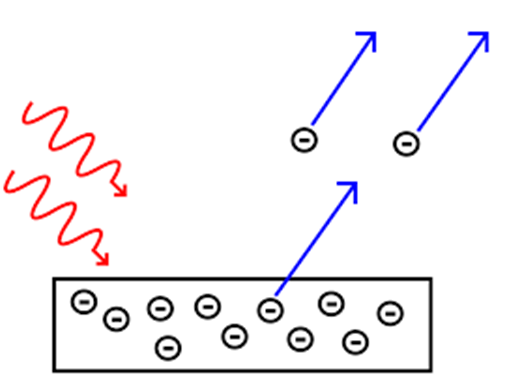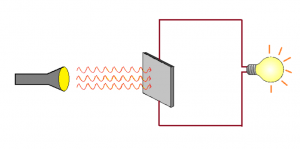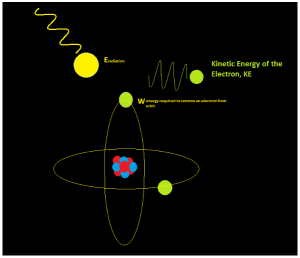# Photoelectric Effect

Photoelectric Effect is one of the most interesting concepts in Physics. The concept of this topic is used to generate electricity directly from the sunlight or the energy of light.

## What is Photoelectric Effect?

When light hits a metal surface it can produce an electric potential. This phenomenon is called a photoelectric effect. This concept is used to generate electricity directly from the energy of light. Solar Panels use this concept in full effect to charge the batteries of the calculators, as a source of power supply in traffic lights and also used as the source of light to light up our homes. Solar energy is one of the reliable sources of energy and it is the largest source of energy on planet earth. The use of solar energy helps in cutting down power costs and to have a healthier environment.

Light of a sufficiently small wavelength can have enough energy to knock out some electrons from a metal surface. This is known as the photoelectric effect. In many ways, this is the opposite of atomic spectral emission where electrons from higher energy states lose energy and emit light. In the photoelectric effect, if a photon of light has sufficient energy, it can raise the electron to a higher energy level and even let it escape the atom’s grasp; in other words, emit an electron. When this happens, a potential difference develops between the atom and the electron, enabling us to use this effect to generate electricity.## Photoelectric Effect Formula

According to the Einstein explanation of the photoelectric effect is:The energy of photon = Energy needed to remove an electron + kinetic energy of the emitted electron

 hν = W + E

Where:

• h is Planck’s constant.
• ν is the frequency of the incident photon.
• W is a work function.
• E is the maximum kinetic energy of ejected electrons: 1/2 mv².### Applications of the Photoelectric Effect

• Used to generate electricity in Solar Panels. These panels contain metal combinations that allow electricity generation from a wide range of wavelengths.
• Motion and Position Sensors: In this case, a photoelectric material is placed in front of a UV or IR LED. When an object is placed in between the LED and sensor, light is cut off and the electronic circuit registers a change in potential difference
• Lighting sensors such as the ones used in smartphone enable automatic adjustment of screen brightness according to the lighting. This is because the amount of current generated via the photoelectric effect is dependent on the intensity of light hitting the sensor.
• Digital cameras can detect and record light because they have photoelectric sensors that respond to different colors of light.
• X-Ray Photoelectron Spectroscopy (XPS): This technique uses x-rays to irradiate a surface and measure the kinetic energies of the emitted electrons. Important aspects of the chemistry of a surface can be obtained such as elemental composition, chemical composition, the empirical formula of compounds and chemical state.For this phenomenon to take place the photons should have the energy greater than or equal to the work function of the metal.

• E≥W
• hf≥W
• f≥Wh
• Where h is the plank’s constant, f is the frequency of the incident photon, and W is the work function of the metal used. The frequency which is equal to w/h is unique for a metal, it varies from metals to metal because the work function of each metal is different from the other. When the photon is absorbed by metal, it transfers a part of the energy to the breaking the electron free from its orbit and rest of the energy is converted into the kinetic energy of the electron itself, therefore the equation for this could be written as,
hν = W + E

Here h is the plank’s constant V is the frequency W is the energy required to remove an electron E is the maximum kinetic energy of the electron Now kinetic energy is given by 12 mv2, therefore, we can find the velocity of the ejected electron by this equation. Look at the following problem, q) Electrons with kinetic energy 6.023 × 10J/mol are ejected from the surface of a metal when exposed to a radiation of the wavelength of 600nm, what is the minimum amount of energy required from the metal atoms.#### Solution:

When the radiation falls on a body some amount of energy is used to knock the electron out of the orbit, rest is used as the kinetic energy of the electron. Therefore,
Eradiation = W + KE

We know kinetic energy per mol lets measure the kinetic energy per electron. There are 6.023 × 1023 electrons in 1 mole of matter, therefore, The kinetic energy of 1 electron would be, 6.023 × 104 6.023 × 1023 = 1 × 10-19J

Let’s measure the energy of each photon,Eradiation = hcλ 6.626 × 10-34 Js × (3 × 108)ms−1600 × 10-9m = 3.313 × 1019J
Now we can equate the two values in the equation and find the unknown work function,
W = Eradiation − KE W = 3.313 × 10-19 − 1 × 10-19 = 2.313 × 10-19 J
Therefore the work function of the metal is found to be 2.313 × 10-19 Joules, the same question can be asked by giving us the work function and asking us to find the velocity at which the electron is ejected, this could be solved with the same equation and the formula for Kinetic energy.

### Important Questions Asked From Photoelectric Effect

Q.1) What is meant by photoelectric effect?
The photoelectric effect refers to the emission, or ejection, of electrons from the surface of, generally, a metal in response to incident light.

Q.2) What is Einstein’s explanation of photoelectric effect?
According to Einstein’s explanation, the photoelectric effect is a phenomenon where electrons are emitted from the metal surface when the light of sufficient frequency is incident upon.

Q.3) Who discovered the photoelectric effect?
The photoelectric effect was discovered by the German physicist Heinrich Rudolf Hertz in 1887.

Q.4) What is photoelectric effect in simple words?
[Refer to Question Number 1]

Q.) Does the photoelectric effect only work on metals?
If you shine a light of high enough energy on to metal, electrons will be emitted from the metal.

Q.5) What did the photoelectric effect prove?
[Refer to Question Number 1 and 2]

Q.6) Why is photoelectric effect important?
The photoelectric effect is important in understanding the quantum nature of light and electrons.

Q.7) What causes photoelectric effect?
[Refer to Question Number 1 and 2]

Q.8) What effect will light intensity have on the photoelectric effect?
Light incident on the surface of a metal in the photoelectric effect causes electrons to be ejected.

Q.9) Why is the photoelectric effect important to modern science?[Refer to Question Number 6]

Q.10) What did the photoelectric effect prove?[Refer to Question Number 1 and 2]

To read about the Nobel Prize-winning explanation by Einstein of the photo-electric effect, download BYJU’S – The Learning App.

#### 1 Comment

1. sibbi

very use full